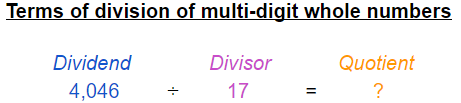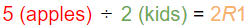# Teach Dividing Multi-Digit Whole Numbers

 Listen to this Lesson:

Fifth grade is an exciting and rich math year. This is when kids need to become fluent in multi-digit whole number addition and subtraction, learn how to add and subtract decimals, and gain a decent understanding of multi-digit whole number multiplication and division.

Since dividing multi-digit numbers is an essential skill that many exercises require, you, as a math teacher or a homeschooling parent, need to make sure your fifth-grade students understand the concept fully before introducing them to more complex mathematical problems. Here’s how you can help them build this foundation and prepare them for practically all future learning with numbers.

## Tips to Teach Dividing Multi-Digit Numbers### Basic Definitions and Terms

Since dividing takes center stage in third grade, it’s smart to start by reminding the children in your classroom what this operation means. One of the simplest and easiest-to-remember definitions is that division means separating a group of things into equal parts. You can use examples from everyday life such as dividing a particular number of beach buckets among a group of children so everyone gets the same number of toys, dividing a certain number of basketball players into teams of five players each, etc. Be sure to emphasize that sharing fairly is key here.

Fifth graders should also be familiar with the terms used in division. Point out that a dividend is a number or amount that needs to be divided or broken down into equal parts. Then, remind your students that a divisor is a number we divide by. Finally, explain to the kids in your class that quotient is the name we use for the answer to a division problem. Writing an example on the whiteboard using different colors can help your students memorize these terms quickly. Here’s an idea:Don’t forget to remind your students that there’s a remainder when the divisor doesn’t divide the dividend evenly. In other words, the remainder is the number or amount ‘left over’ after the division process is completed. Use this simple example to help them figure out what this means:Explain to the children that when 5 apples need to be equally divided among 2 kids, 1 apple will remain unshared. This is in fact the remainder. If the division problem is solved correctly, the remainder will be less than the divisor (1<2 in the example above).

### Standard Algorithm for Multi-Digit Number Division

If you’re confident that your fifth-grade students know how to confidently add, subtract, and multiply multi-digit whole numbers, you can introduce them to the standard algorithm for multi-digit whole number division. Begin by highlighting that the standard algorithm is the most commonly used method for solving division problems, which is also the case with multi-digit whole number addition, subtraction, and multiplication.

It’s also good to mention that dividing multi-digit numbers isn’t very different from dividing by a one-digit number. In both cases, breaking up the division problem into a series of smaller and therefore easier division steps is crucial.

Okay, it’s now time to guide the children in your class through the process of dividing multi-digit whole numbers step by step.

#### Step 1: Divide

Explain to your students that division problems with multi-digit divisors can’t be solved by starting with only the first digit of the dividend (this works only with one-digit divisors). Since the divisor is bigger than the first digit of the dividend, they need to take the first two, three, or more digits of the dividend, i.e. add one or more place values. Help them understand what you’re talking about with this example:

17548

Explain to the children that since they’re dealing with a two-digit divisor (17), they can’t start by asking themselves: “How many times does 17 fit into 5 (the first digit of the dividend)?” They should take into account the first two digits (54) instead and ask “How many times does 17 fit into 54 without going over it?”

Point out that this is when things get a bit difficult as multiples of 17 aren’t on the multiplication table. Tell them that that’s why they need to estimate or make a good guess. Let them know that some complex division problems might require a bit of trial and error, i.e. making multiple guesses before figuring out the answer. In this case, 17 goes into 54 3 times without going over it.

3
17548

Don’t forget to mention that the answer needs to be written above the tens place because the first digit was skipped.

#### Step 2: Multiply

Explain to your students that now they need to multiply the answer they got in the previous step and the divisor (3 × 17). After that, they need to write the product under the two digits they grouped together (54).

3
17548
51
____

#### Step 3: Subtract

Instruct your fifth graders to subtract 51 from 54 and write the answer below 51 like so:

3
17548
– 51
____
3

Now’s a great time to let your students know that they should always make sure the remainder is less than the divisor (3<17). If the remainder is equal to or larger than the number they’re dividing the dividend by, that means they’ve made an underestimation in step 1. For example, if they estimated that 17 goes into 54 twice, they’d end up with a remainder of 20, which is obviously bigger than 17. In other words, 17 can fit into the remainder once, which is why the correct answer is 3.

#### Step 4: Bring Down

This is when you need to instruct your students to bring down the next digit of the dividend to join the remainder. In this case, that’s 8.

3
17548
– 51
____
38

#### Step 5: Repeat

Point out to your fifth graders that they need to repeat the first three steps. If the dividend had 4 or more digits, they’d need to repeat the process until they’d brought down the last digit of the dividend.

32R4
17548
– 51
____
38
– 34
____
4

The quotient of 548 ÷ 17 is 32 with a remainder of 4.

Explain to your students that they can verify the answer using multiplication. Write this formula on the whiteboard:

(Divisor x Quotient) + Remainder = Dividend

(17 x 32) + 4 = ?
(544) + 4 = ?
544 + 4 = 548

To help the children in your class get the hang of dividing multi-digit whole numbers more easily and quickly, advise them to round the numbers up. For instance, if they need to figure out how many times 49 can fit into 512, they can approximate 49 to 50 and 512 to 500. Figuring out how many times 50 can go into 500 is so much easier (10 times). Be sure to warn them that this might not always give them a precise answer, though. They’ll know they’ve made a mistake if the remainder is equal to or bigger than the divisor.

## Practice Dividing Multi-Digit Whole NumbersPractice makes perfect, right? 100%! To help your fifth graders become fluent in dividing multi-digit numbers, have them solve word problems and check the answers using multiplication. This will help them understand the connection between multiplication and division, i.e. realize that division is the opposite of multiplication.

You can also assign your students to play games. To keep them entertained and willing to solve multi-digit whole number division problems, you can have them play a math version of Who Wants to Be a Millionaire. Prepare 10-20 division problems with different difficulty levels (feel free to change this number if necessary) and offer 4 potential answers for each of the problems. Instruct your students to pick only one answer per problem. The student who will manage to solve all the problems correctly will win the prize of 1 million (imaginary) dollars. To make the game more fun, give the kids 15-20 minutes to figure out the answers (adjust the game duration if needed).

In case nobody solves all 10 or 20 problems accurately, go through them together and address all confusions.

## Before You Leave…

We’re confident that each of these tips and activities will give you a good idea of how to help your fifth-grade students develop proficiency with dividing multi-digit whole numbers. If you’re looking for more math resources for kids of all ages, be sure to sign up for our email today, get a membership at MathTeacherCoach, and/or check out our blog where you’ll find tried-and-true teaching advice and games that will help you design and teach fun and successful classes.